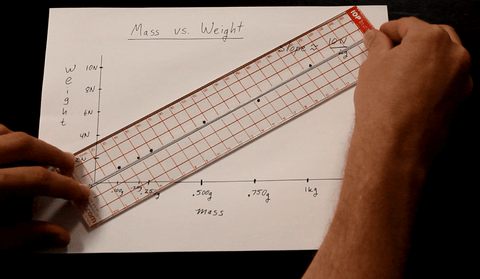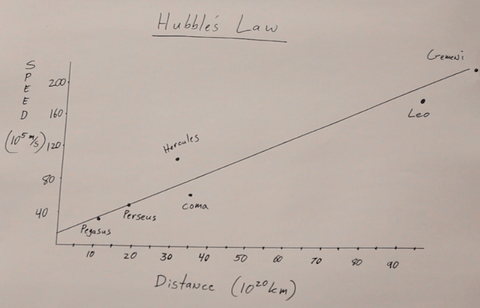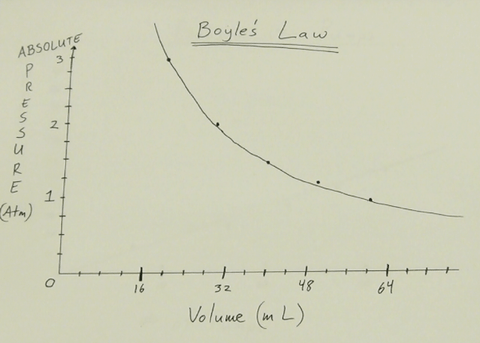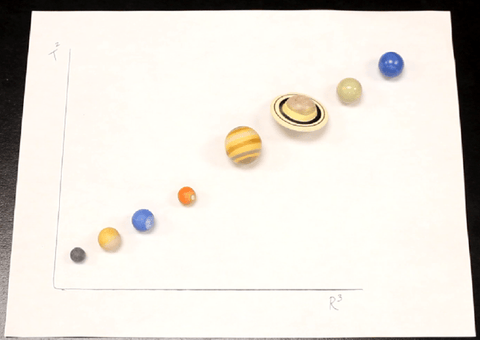# The Importance of the Best Fit Line

Introduction

One of the most important skills that you will learn in physics class is drawing a best fit line. Usually what happens is you take two related variables and chart how they relate to each other. For example, mass vs. weight which of course are directly related to one another. As the mass increases, its weight increases, at least in a gravity field.

A proper best fit line will have the same amount of points above your line as below your line. This is not a skill that can be learned using Microsoft Excel, which rushes you to the end without thinking. It is important that we give students a chance to think about why best fit lines are the way they are. By the way, there is no access to Excel graphing programs on the AP Physics Test!Tips for Graphing Scientific Data

The independent variable (in this case the mass) should be graphed on the X-Axis, and the Y the dependent variable goes on the Y-Axis. We control the independent variable, and can change it independent of other changes, but we have no control over the dependent variable. It depends on the conditions of the experiment.

It is true that not all relationships are linear, but there are techniques that can be used to make uneven powers of exponents come out straight (see Non-linear Relationships below).Students and teachers often get confused whether we graph is y vs x or x vs y – the latter is wrong. I would like to clear this up with a mnemonic. V can stand for vertical and S for sideways. Then it would be easy and correct to write y VS x which helps us remember that vertically graphed variable is said first. For example, consider "Rain VS. Latitude." You can see that the latitude is the horizontally-graphed, independent variable because scientists can independently choose which latitude to measure, but we have no control over the amount of rain. Thus the dependent variable, graphed vertically, is mentioned first.

Activities and Ideas

One of the first lab activities I do with my physics students is to plot Celsius vs. Fahrenheit on an XY plane. This helps the students to relate the important temperatures like freezing or the human body temp to train them to draw a best fit line. Since they are graphing by hand, it is guaranteed that there will be some error in plotting the points. The slope is the familiar 9/5 from the C to F relationship of F = 9/5 C +32 that you might have learned in a chemistry class. You can derive this relationship using y = mx + b.

Other famous times when a best fit line came in handy…when the Hubble's relationship between distance and speed for galaxies. The further away a galaxy is, the faster it is moving. This is actually a linear fit—no squaring required! It is easy to appreciate that this is consistent with a universe expanding in 3D. Imagine two points on a balloon, let's call them "The Milky Way" and "The Leo Galaxy." As the radius of the balloon increases the distance between these points increases. The further apart they are, the faster they are moving apart.

Perhaps a more down to earth example might be the relationship between foot size and height. Of course we expect that people with larger feet will be taller. But these two variables are correlated with a high degree of variance which can lead to an interesting scatter plot. Also, students enjoy this lab.Derive Fahrenheit to Celsius conversionHubble's Law

The Best Fit Line Ruler

When I made the graphs in the video that accompanies this article I used real data from experiments that I actually did that day. I found that the Best Fit Line Ruler made the graphing go much more smoothly. The ruler will help you draw your line between the points you have plotted and make sure that you have the same amount above as below.

Also, the best fit line will not be messed up, particularly not when you use this tool. I have always had trouble with neatness and spacing, and I was surprised by how well it cleaned up that issue. Many times students will not space their hash marks evenly and it makes the data come out skewed, which can be frustrating. I found myself repeatedly using the guidelines to help my spacing. You will be impressed with how much you enjoy using it.You can see that the fact that is clear helps enormously. You can see the trend more easily, especially in scattered distributions. This can be very helpful in statistics as well as science, and it might convince students to abandon their obsession with connecting dots.

Making Linear Graphs of Uneven Powers

One of the most important skills that accompanies best fit line drawing is knowing how to get uneven powers to come out straight. For example, the period, T, of a pendulum is supposed to be T = 2π √ (length/gravity). But if you square the period and graph it vs the length, then you will see a straight line, which is an experiment that I advise you to do.

An inverse relationship can be graphed with a positive slope, too. For example, Pressure vs. Volume. In this case as the volume increases, the pressure decreases. In order to make the graph come out as a positively sloped "y = mx + b" I recommend graphing the inverse of the volume on the X-axis. Now the graph becomes Pressure = slope * (volume)^ -1 + b. The intercept, b, should be zero.

When it comes to pressure experiments, most gauges will ignore the atmospheric pressure and call that zero. We have to add 14 PSI or 105 Pascals to this value to adjust it to "absolute pressure."

Perhaps the most famous best fit line story of all time is Kepler's 3rd Law. The planets move in ellipses, and they are faster when they are close to the sun and slower when they are further away. That's the law… "The square of the period of any orbit divided by the cube of the semi-major axis is constant for all planets." (An ellipse has two diameters or axes, and the larger one is called the major axis. Half of that is the semi-major axis.) For most planets, the orbits are within 2% of being a perfect circles, so you can be fairly accurate by simplifying the law to T2/R3=constant.An uneven power can be graphed straightAn inverse graph with a positive slopeBecause of the vast distances, it is not easy to get all the planets on the same graph unless you utilize a logarithmic scale. Some of your students might not be ready for this. Instead, you can more easily try graphing the Kepler Relationship for objects that have fairly close periods, such as the moons of Jupiter or the moons of Saturn. T2/R3=constant will still hold for these orbiting bodies, the only difference is that the constant is now a different constant. This was a problem that came up on the 2005 AP Physics C Test.

Conclusion

Performing a best fit line drawing by hand is an important skill that is expected of our students. The logic is that if they truly understand the relationships between variables, then they will be able to decide how to graph them so that their relationship is displayed on a linear graph.

The best fit line ruler is an improvement on typical rulers, no rulers, or using Microsoft Excel. The value of the product is found in both its convenience and its ability to remind us of skills that we should be focusing on developing in our students.James Lincoln

Tarbut V' Torah High School

Irvine, CA, USA

James Lincoln teaches Physics in Southern California and has won several science video contests and worked on various projects in the past few years. James has consulted on TV's "The Big Bang Theory" and WebTV's "This vs. That" and the UCLA Physics Video Project.

Contact: James@PhysicsVideos.net

Collin Wassilak